﻿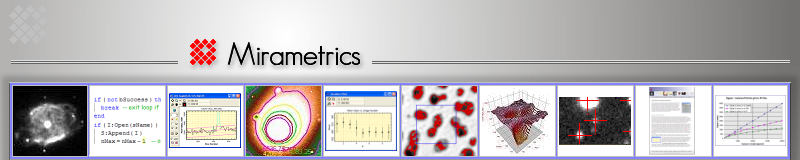1-D Data Visualization in Mira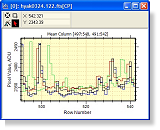This Brief describes the various types plots  that can be created by Mira for visualizing 1-D data (also see the 1-d Fit package built into Mira MX x64). Mira's 1-D plotting architecture was designed to efficiently and effectively work with data sets consisting of many points, many images, and many series. Mira can create representations like step-line plots and x/y error bars. Plots can also be printed on any printer supported by Windows to obtain publication-quality hardcopy.

Although most plots are made from data in an Image window, plots can also be generated from tabular measurements and by using the extensive and versatile collection of plotting functions in the scripting module. Not discussed here are topics like scripted plots, working with plot data, plot commands, toolbars, plot attributes, etc.

## Plot Types

The table below describes plotting capabilities of various Mira platforms. Not all features are in all platforms, and such cases are noted.  Pictures...

### Column Profile

Plots the intensity of a single column or all columns in a region of interest, for 1 image or an image stack. The single column intensity may also be the mean, median, or sum of all columns in the region of interest.

### Row Profile

Plots the intensity of a single row or all rows in a region of interest, for 1 image or an image stack. The single row intensity may also be the mean, median, or sum of all rows in the region of interest.

### Line Profile

Plots the intensity along a drawn line between two points. Optionally, the starting or ending point may be auto-centroided to the moment-weighted local maximum or minimum sub-pixel position. Additional intensity series may be added to the plot using lines exactly parallel to or displaced from the first line.

Plots the radial intensity profile in all directions from a sub-pixel positioned center point. Optionally, the center point may be auto-centroided to the moment-weighted local maximum or minimum sub-pixel position. Optionally, a Gaussian + Constant model may be fit to the sample data and the results reported for estimation of FWHM, Peak intensity, and background luminance.

### Histogram Plot

Shows the frequency distribution of image values inside a region of interest. The plot may be made for one image or an entire image stack may be plotted on the same set of axes.

### Bit Histogram Plot

Plots the frequency of bit values inside a region of interest. The plot may be drawn for one image or an image stack. (Mira Pro and Mira MX)

### Scatter Plot

Plots discrete points from tables containing measurement results. The columns to plot are selected from the table. (Mira MX)

### Pixel Series Plot

Plots multiple plot series showing  the pixel value at a given location for the members of an image stack. (Mira Pro and Mira MX).

### Custom Plots

Plots can also be generated by the MX Script and Pro Script modules. In addition to the plot types above, scripted plots may contain any number of points in any number of series with any combination of lines, points, error bars, colors, attributes, etc.

### Data Fitting

Mira MX provides an outstanding tool for analyzing 1-dimensional data using polynomial regression. Features include iterative sigma clipping, forced coefficient values, selectable data series, auto-rejection, manual deletion and weighting, and more.  More

## Examples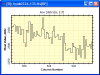### A Simple Plot

This shows a simple plot along a single column of an image. The Plot Toolbar (at the top of the window in other pictures, below), is disabled in this view.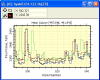### Overplot of Column Intensity Plots from 5 Images

The Image Window displayed a stack of 5 images. A single button click plotted the same column in each image. The colors assigned to the 5 plot series can be specified. At the top of the window is shown the Plot Toolbar provided with Mira Pro.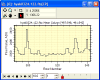### Animating the 5 Column Plots (from above)

Using the same data as above, the Plot Windows was switched to Animate mode. This shows 1 plot series at a time which can be stepped, selected, or animated in various ways. At the top of the window is shown the Plot Toolbar provided with Mira MX.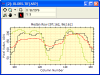### Comparing Plots on the Same Axes (Mira Pro and MX)

Separate plots were created for a single row slice, a median row slice and a mean row slice (each in a different window). The Single Row and Median Row plots were appended to the Mean Row plot using the plot copy+paste commands in Mira Pro and Mira MX.### Comparing Histograms of several images (Mira Pro and MX)

This example plots the histogram of the same region of interest in 5 images. The images were opened as an image stack, then the histogram plot button clicked once. All plots were originally drawn in black. The plot for image 2 was changed to red as a reference for comparison with the other histograms. [Changing attributes of individual plot series is a feature of  Mira MX.]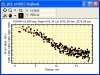### Radial Profile Plot with Fit

This example shows a standard Mira feature of all platforms: the radial profile plot. This type of plot is important for characterizing the point spread function of an image. Optionally, a Gaussian + Constant model is fit to the data and is used to estimate the FWHM, Background, and Peak value of the profile data. Even with very low signal to noise ratio data, as shown here, Mira's fit is robust and the estimated parameters are accurate. In addition, the visual drawing of the fit through the data provides an unambiguous check on the validity of the estimated parameters.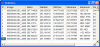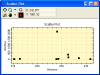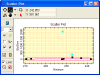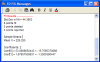### Scatter Plot with Polynomial Fit (Mira MX)

This example shows the result of making, plotting, and performing regression analysis on the results of image measurements. Staring with a table of measurements,  6 button clicks and about 10 seconds selected and plotted the target data columns and produced the regression results.

• The picture at top shows the accrual of 10 statistics measurements of regions of interest on the same image. This uses a Mira Report window.
• Using the tabulated measurements, the second picture shows a scatter-plot of values in the Standard Deviation column (Std Dev) versus values in the Minimum Value column. Clearly, there is at least 1 high outlier that probably sampled a radiation event or other artifact.
• The third picture shows a linear fit (2 terms) with sigma rejection enabled. This employed the 1d Fit package which uses the toolbar shown on the left window border. Two outlying points were rejected above 2.5 sigmas (blue).
• The bottom picture shows messages from the 1d Fit Package in a Mira Text Window.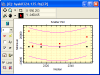### Scatter Plot with Polynomial Fit (Mira MX)

This example shows a scatter plot with a 3-term polynomial fit. The data were generated by a script that computed the regional minimum value from several images. The script created and populated the Plot Window. After the script terminated, in Mira MX, the 1d-Fit toolbar was opened and the fit computed interactively on the data. This fit uses iterative sigma clipping. The upper and lower purple curves show 3 sigma deviations from the fit, which shows no points rejected. The black and red points show two different plot series resulting from the two groups of images being compared.### Custom Plot Created by a Script

This illustrates the versatility of plots generated by Mira scripts module. This plot mixes points symbols, step-lines, x and x-y error bars, etc.

﻿
 Main Links Home Products Mira Blog Store Briefs Tech Notes Support Who uses Mira? Purchasing Info Press Web Tools Contact Overview of Mira Dealers General Info Legal Info Products Mira MX x64 Mira Pro x64 Mira Pro Mira AL Scripting in Mira File Event Scripting Feature Comparison Source Extraction Mira vs IRAF